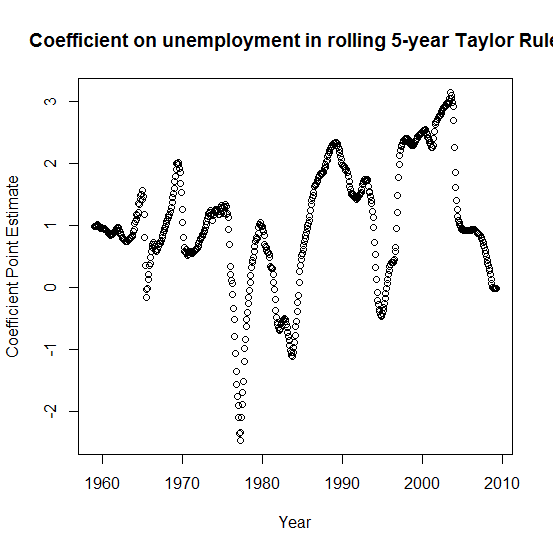### How has the Taylor Rule performed?

6/29/2014 03:41:00 PM
Tweetable

The Taylor Rule is a theoretical equation that supposedly predicts what the Federal Reserve will set the nominal "fedfunds" interest rate at, depending on the inflation rate and unemployment rate at the time. Some version of it has reigned at the heart of all monetary macro models since the late 1980s. The equation looks like this: $$r=\pi+\alpha\left(\pi-\pi^*\right)+\beta\left(u^*-u\right)+\gamma$$ where $r$ is the fedfunds rate set by the Federal Reserve, $\pi$ is the inflation rate, $\pi^*$ is the target inflation rate, $u^*$ is the estimate for "full employment" also known as the "non-accelerating inflation rate of unemployment" (NAIRU), $u$ is the actual unemployment rate, and $\alpha,\beta,\gamma$ are constants picked by the Fed. I found data for $r$, $\pi$, $u$, and $u^*$ on FRED. Recently, the Fed has announced an inflation target of 2 percent, though as best as we can tell this is what it has always been. Because there's no variation in $\pi^*$ this leaves our model technically underidentified, but we can back out the estimates by rewriting the equation as $$r=\left(1+\alpha\right)\pi+\beta\left(u^*-u\right)+\gamma-\alpha\pi^*$$ which can is straightforward to estimate with OLS. The Taylor Principle says that $\alpha\gt 0$, and we would generally expect $\beta\gt 0$, if the Fed desires to minimize unemployment as much as possible.

I've just run this regression 603 times, estimating Taylor Rules over every 5 year period from 1959 to present, on a monthly basis. I've extracted the coefficients from the OLS and plotted them below:Estimates of $\left(1+\alpha\right)$Estimates of $\beta$I won't claim that my regressions are necessarily the most robust analysis, but it certainly seems that the notion of the Fed following any kind of Taylor rule is almost entirely apocryphal. In reality, the coefficients aren't even consistently positive over 5 year periods.

Or, to put this into terms John Taylor can understand, the Taylor Rule is not Lucas-robust. Not by a longshot.

I will leave it to my readers to interpret what the coefficients say about economic history of the United States. (For example, where's the easy money policy that caused thestagflation in the 1970s? Where's the tight money policy that caused the disinflation of the 1980s?)

As always, you're free to review my data (also need this) and my R script file (some editing required).Nick Rowe 7/14/2014 03:50:00 PM
Good post.

If the central bank is targeting a fixed rate of inflation, the role of the alpha coefficient being positive is as a threat strategy. As long as that threat is credible, expected inflation will not deviate from target, and we would never observe the threat being carried out.

I think that what we are actually observing in the data is a mix of three things:

1. Changing inflation targets over time.

2. The correlations between the Fed's response to shocks and the economies response to those same shocks

3. The Fed's learning over time that it is making mistakes in estimating the natural rates r* and u*.